Given a straight segment of track of length, add a small segmentso that the track bows into a circular Arc. Find the maximum displacementof the bowed track. The Pythagorean Theorem gives(1)

Butis simply, so(2)

Solving (1) and (2) forgives(3)

Expressing the length of the Arc in terms of the central angle,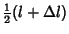(4)

Butis given by(5)

so pluggingin gives(6)(7)

For,(8)

Therefore,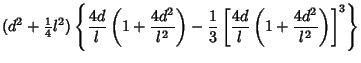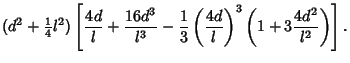(9)

Keeping only terms to order,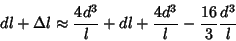(10)(11)

so(12)

and(13)

If we takeand1 foot, then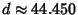feet.